# How to create an Excel Balance Sheet

You can easily create an Excel Balance sheet using vertical or horizontal format, depending on your choice. Creating an Excel balance sheet can provide users with readymade tables and formulas. Excel reduces calculation mistakes and saves labor with endless columns and rows. You have to enter data alongside the formulas, and you will get the desired results. It will be able to show liabilities and assets that the company owns.

Before creating Excel Balance Sheet, remember that a balance sheet is made of two sections connected with the equation.

Assets = Liabilities + Assets

Assets are organization resources that generate future returns like buildings, receivables, land, etc. The assets are divided into two categories non-current assets and current assets.

• Current assets refer to assets that are liquid and hold a year or less lifespan. These assets can be easily liquidated and turned into cash. Examples include inventory, account receivables, cash, prepaid expenses, etc.
• Non-current assets: – Stands for assets that are not liquid and have a long lifespan of more than one year. They can be converted into liquid cash easily. They include vehicles, machinery, equipment, land, etc. They also consists of assets that are not tangible like copyright, patents, goodwill, and contract.

Liabilities are the organization’s obligations, whether to another company or an individual. They include loans, payables, etc. It can either be current and non-current liabilities.

• Current liabilities: – this refers to liabilities with a discharge one year term and below. Examples include wages, rent, short-term loans, account payables, utilities, taxes payable, etc.
• Non-current liabilities: – It is also called long-term liabilities. They include bank debts, differed taxes, pension funds liabilities, and principal on bonds. They are obligated to be discharged after a one year term.

Equity can be referred to as the value in which a company’s shareholders holds after selling all the company’s assets, and all the liabilities have been paid off. Equity is a residual of the company after subtracting liabilities from assets. Equity also includes retained earnings and capital stock.

## How to create a balance sheet using a vertical format

A vertical balance sheet its columns are arranged vertically. Where it consists of two tables, one is on top of the other table. That is the assets column at the top and the liabilities and equities table below it. For example, in this example, G Moody has the following assets, capital, and liabilities as on 31 Dec 2022:

Sh in dollars

Creditors 18,160

Equipment 51,520

Motor Vehicle 25,160

Stock 26,680

Debtors 19,480

Cash at bank 27,040

Capital 131,720

Draw up the balance sheet for the organization.

You can construct a vertical balance sheet by following these steps.

1. In an open workbook, start typing the balance sheet title at the beginning and enter the date. Click cell A1 and type G Moody Balance Sheet as at 31 Dec 20222. Select cell A1:D1 and Click on the Home tab of the main menu ribbon.

3. In the Alignment group, click on the Merge & Center options and choose Merge Cells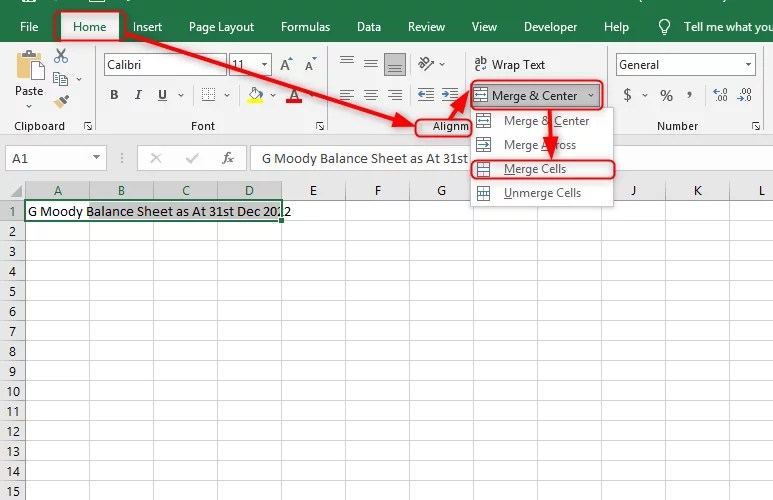4. In cell B2, make a heading named ASSETS below cell (B3). Insert a subheading for Current Assets

5. Below the subheading from the example, you will enter the current asset types. In this example, they are Stock, Cash at Bank, and Debtors and record the value of the assets on the right.

6. Ensure the asset value are written in Accounting number format. Select cell C4:C6. Click on the Home tab of the main menu ribbon. In the Number group, click on the Number Format options then Accounting number format. Then press OK.7. In cell B7, enter the Total current assets. Record the value of the assets on the right by using the sum function. Select cell C7 input function =SUM (C4:C6). Where C4:C6 are current assets.8. In cell B9, enter the non-current assets below and enter the types. In this example, they are Equipment and Motor vehicles, and record the assets value on the right.

9. In cell B12, enter the Total Non-current assets. Record the assets value on the right by using the sum function. Select cell C12 and input function =SUM (C10:C11). Where C10:C11 are current assets.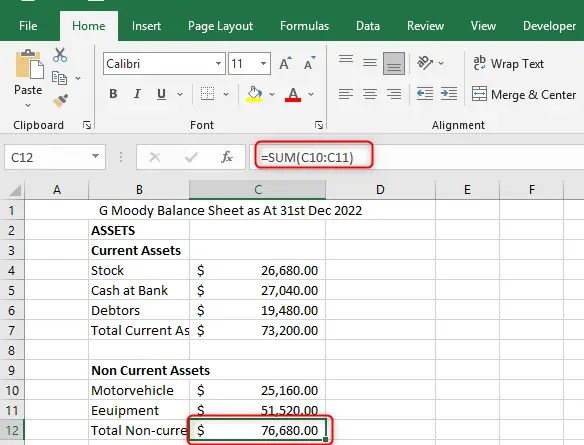10. Record assets value on the right by using Excel Formula. In cell B14, enter the Total assets. Select cell C14 and input function =C7+C12. Where the total assets, add the Total current assets and Total Non-current assets.11. In cell B15, make a heading named LIABILITIES & EQUITY below cell (B16). Insert a subheading for Current liabilities

12. Below the subheading from the example, you will enter the current liabilities types. In this example, they are creditors and record the liabilities value on the right.

13. Ensure the asset value are written in Accounting number format. Select cell C17. Click on the Home tab of the main menu ribbon. In the Number group, click on the Number Format options and choose Accounting. Then press OK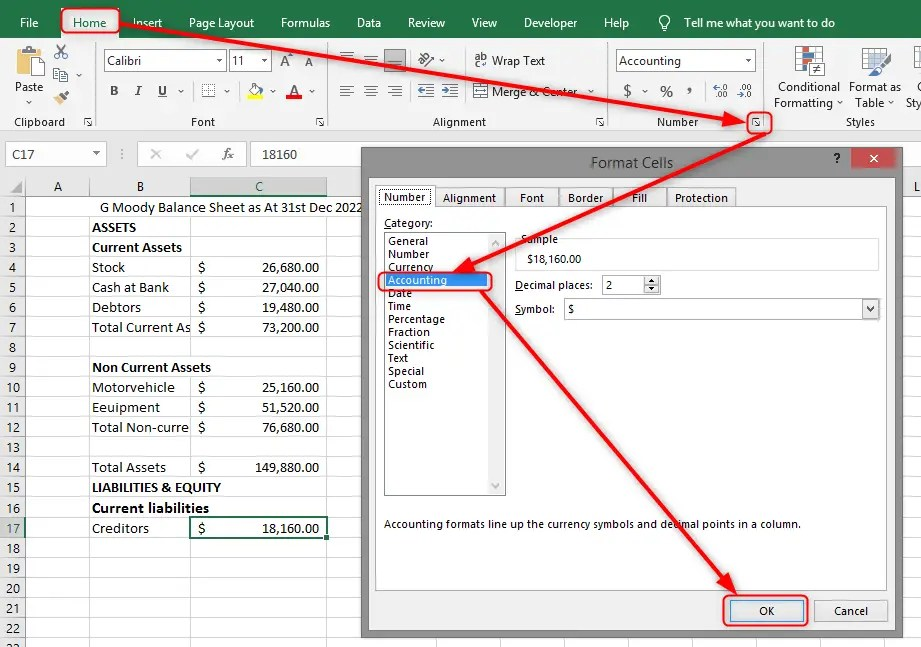14. In cell B18, enter the Total current liabilities. Record liabilities value on the right by using the sum function. In this example, the value will be 18860 dollars since we have one current liability creditor.

15. In cell B20, enter the long-term liabilities below, give the types and record the liabilities value on the right.

16. Below it, enter the Total long-term liabilities. Use the sum function or Excel formula to record the liabilities value on the right. On the next cell, record the total liabilities. Calculate it by adding current liabilities with long-term liabilities. In this example, we don’t record long-term liabilities, total long-term liabilities, and total liabilities since we have only one current liability.

17. In cell B20, enter the Equity below and enter the types. In this example, we have capital only, so we record the value on the right in accounting number format.

18. Below it, enter the Total Equity. Use the sum function or Excel formula to record the Total Equity value on the right. In this example, we don’t record total equity since we have only one equity.

19. In cell B23, enter Total liabilities and Equity. Record Total liabilities and Equity value on the right using the Excel formula =C21+C18. Where we Total Equity to Total Liability. You are done. There you have your vertical format of the Balance Sheet.Note: your Total ASSETS=TOTAL LIABILITIES & EQUITY to show that you have been able to create the balance sheet correctly.

## How to create a balance sheet in Excel using a vertical format

Using the above example, you can create a horizontal format balance sheet. You have to follow the following steps

1. First, start by typing the title of the balance sheet and enter the date. Click cell A1 and type G Moody Balance Sheet as at 31 Dec 20222. Select cell A1:D1 and Click on the Home tab of the main menu ribbon.

3. In the Alignment group, click on the Merge & Center options and choose Merge Cells4. Then since it is a vertical format, you make two columns for Assets and Liabilities5. Under the Assets column, cell (A3) insert a subheading showing the Current Assets

6. Below the subheading from the example, you will insert the current assets. In this example, they are Stock, Cash at Bank, and Debtors and record the assets value on the right.

7. Ensure the asset value are written in Accounting number format. Select cell C4:C6. Click on the Home tab of the main menu ribbon. In the Number group, click on the Number Format options and choose Accounting number format. Then press OK.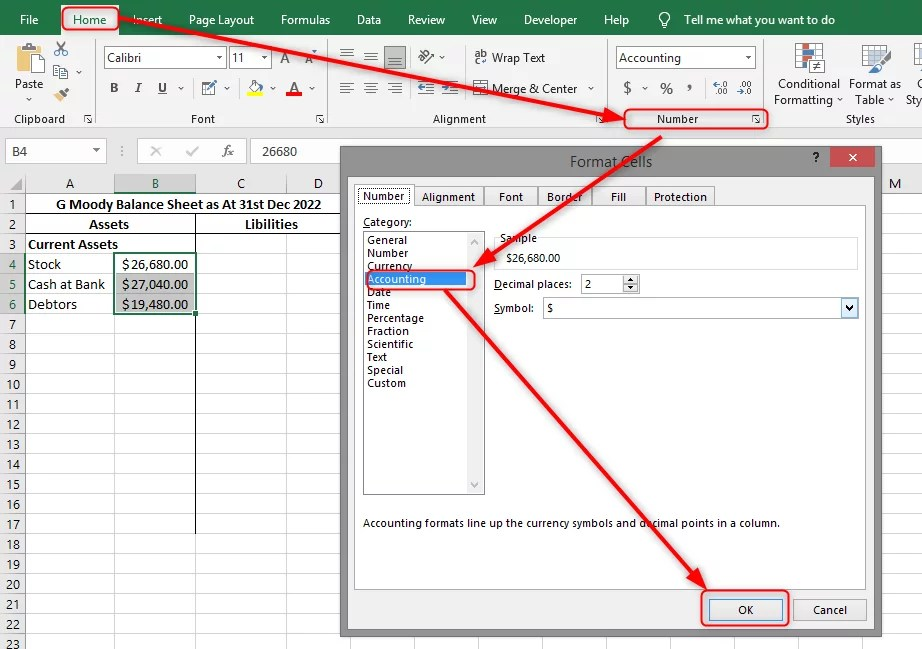8. In cell A7, enter the Total assets. . Record the assets value on the right using the sum function. Select cell B7 input function =SUM (B4:B6). Where B4:B6 are current assets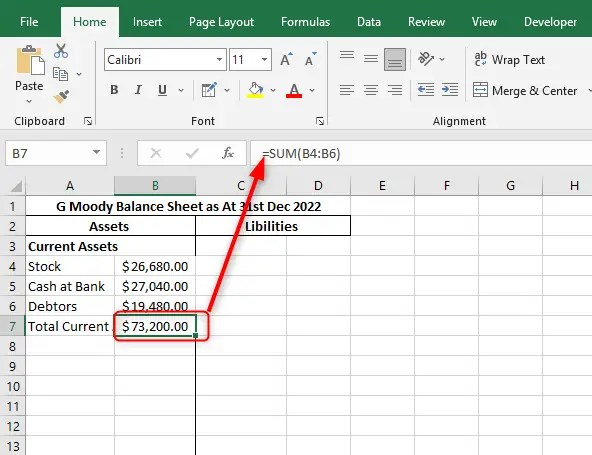9. In cell A9, enter the non-current assets below enter the types. In this example they are Equipment and Motor vehicle and record the assets value on the right.

10. In cell A12, enter the Total Non-current assets. Record the assets value on the right by using the sum function. Select cell B12 and input the function =SUM (B10:B11). Where B10:B11 are current assets.11. In cell A14, enter the Total assets. Record assets value on the right by using Excel Formula. Select cell B14 and input function =C7+C12. Where the total assets are Total current assets added with Total Non-current assets.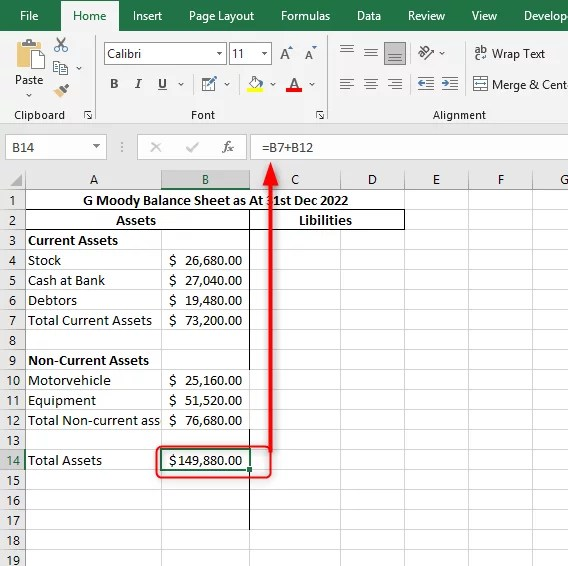12. Under the liability column, select cell C3. Insert a subheading for Current liabilities.

13. Below the subheading from the example, you will enter the current liabilities types. In this example, they are creditors and record the liabilities value on the right. Ensure the asset value are written in Accounting number format.

14. In cell C5, enter the Total current liabilities. Record liabilities value on the right by using the sum function. In this example, the value will be 18860 dollars since we have one current liability creditor.15. In cell C7, enter the long-term liabilities below, give the types and record the liabilities value on the right.

16. Below it, enter the Total long-term liabilities. Use the sum function or Excel formula to record the liabilities value on the right. On the next cell, record the total liabilities. Calculate it by adding current liabilities with long-term liabilities. In this example, we don’t record long-term liabilities, total long-term liabilities, and total liabilities since we have only one current liability.

17. In cell C7, enter the Equity below and enter the types. In this example, we have capital only, so we record the value on the right in accounting number format.

18. Below it, enter the Total Equity. Use the sum function or Excel formula to record the Total Equity value on the right. In this example, we don’t record total equity since we have only one equity.

19. In cell C10, enter Total liabilities and Equity. Record Total liabilities and Equity value on the right using the Excel formula =D5+D8. Where we Total Equity to Total Liability. You are done. There you have your horizontal format of the Balance Sheet.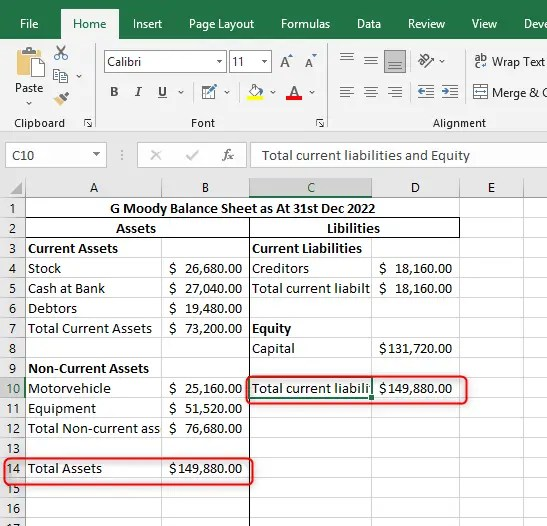Note: The general principle of accounting requires that the total values in the Assets column should equal the total of Liabilities and Equity.